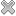# Courses / Module

Toggle Print

##FOUNDATIONS OF DATA SCIENCE 1

Module code: HM820DS
Credits: 5
Semester: 1
Department: HAMILTON INSTITUTE
International:Coordinator: Prof. Ken Duffy (HAMILTON INSTITUTE)Overview

The aim of this module is to introduce the range of applied mathematical and statistical techniques needed to understand data science algorithms, forming a foundation for PhD research on the development of new methods. Syllabus: 1. Mathematical foundations: multivariate calculus, linear algebra, probability theory, 2. Mathematical modelling: asymptotic analysis, uncertainty quantification, 3. Networks, 4. Optimisation, 5. Computational programming, 6. Statistical inference, 7. Statistical computing.Learning OutcomesTeaching & Learning methodsAssessmentRepeat optionsPre-RequisitesTimetable